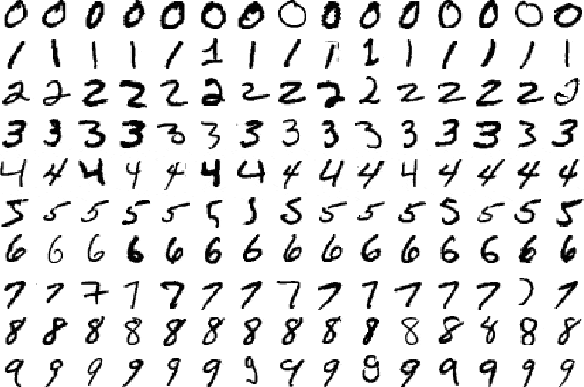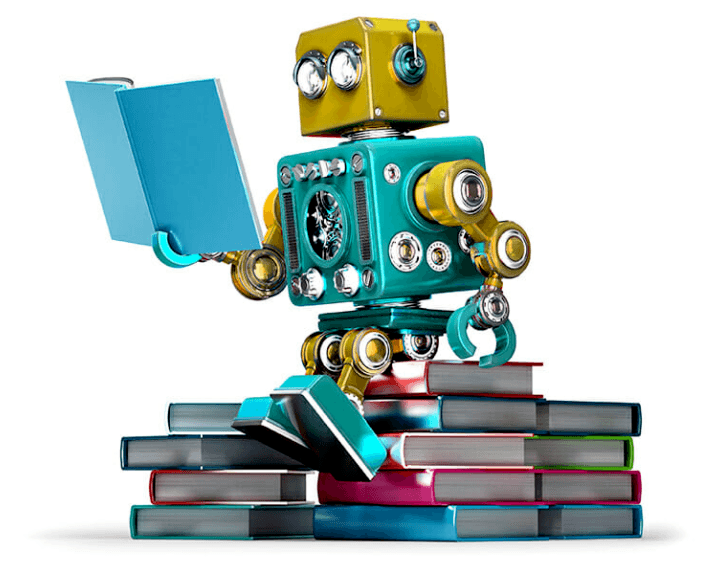#.

Sometimes we encounter problems for which it’s really hard to write a computer program to solve. For example, let’s say we wanted to program a computer to recognize hand-written digits:Source: MNIST handwritten database

You could imagine trying to devise a set of rules to distinguish each individual digit. Zeros, for instance, are basically one closed loop. But what if the person didn’t perfectly close the loop. Or what if the right top of the loop closes below where the left top of the loop starts?A zero that’s difficult to distinguish from a six

In this case, we have difficulty differentiating zeroes from sixes. We could establish some sort of cutoff, but how would you decide the cutoff in the first place? As you can see, it quickly becomes quite complicated to compile a list of heuristics (i.e., rules and guesses) that accurately classifies handwritten digits.

And there are so many more classes of problems that fall into this category. Recognizing objects, understanding concepts, comprehending speech. We don’t know what program to write because we still don’t know how it’s done by our own brains. And even if we did have a good idea about how to do it, the program might be horrendously complicated.

So instead of trying to write a program, we try to develop an algorithm that a computer can use to look at hundreds or thousands of examples (and the correct answers), and then the computer uses that experience to solve the same problem in new situations. Essentially, our goal is to teach the computer to solve by example, very similar to how we might teach a young child to distinguish a cat from a dog.

## What is Machine Learning? — Definition

The field itself: ML is a field of study which harnesses principles of computer science and statistics to create statistical models. These models are generally used to do two things:

1. Prediction: make predictions about the future based on data about the past
2. Inference: discover patterns in data

Difference between ML and AI: There is no universally agreed upon distinction between ML and artificial intelligence (AI). AI usually concentrates on programming computers to make decisions (based on ML models and sets of logical rules), whereas ML focuses more on making predictions about the future. They are highly interconnected fields, and, for most non-technical purposes, they are the same.--   --   --

## What’s a statistical model?

Models: Teaching a computer to make predictions involves feeding data into machine learning models, which are representations of how the world supposedly works. If I tell a statistical model that the world works a certain way (say, for example, that taller people make more money than shorter people), then this model can then tell me who it thinks will make more money, between Cathy, who is 5’2”, and Jill, who is 5’9”.

What does a model actually look like? Surely the concept of a model makes sense in the abstract, but knowing this is just half of the battle. You should also know how it’s represented inside of a computer, or what it would look like if you wrote it down on paper.

A model is just a mathematical function, which, as you probably already know, is a relationship between a set of inputs and a set of outputs. Here’s an example:

f(x) = x²

This is a function that takes as input a number and returns that number squared. So, f(1) = 1, f(2) = 4, f(3) = 9.

Let’s briefly return to the example of the model that predicts income from height. I may believe, based on what I’ve seen in the corporate world, that a given human’s annual income is, on average, equal to her height (in inches) times 1,000. So, if you’re 60 inches tall (5 feet), then I’ll guess that you probably make \$60,000 a year. If you’re a foot taller, I think you’ll make \$72,000 a year.

This model can be represented mathematically as follows:

Income = Height × \$1,000

In other words, income is a function of height.

Here’s the main point: Machine learning refers to a set of techniques for estimating functions (like the one involving income) based on datasets (pairs of heights and their associated incomes). These functions, which are called models, can then be used for predictions of future data.

Algorithms: These functions are estimated using algorithms. In this context, an algorithm is a predefined set of steps that takes as input a bunch of data and then transforms it through mathematical operations. You can think of an algorithm like a recipe — first do this, then do that, then do this. Done.

Machine learning of all types uses models and algorithms as its building blocks to make predictions and inferences about the world.

## What exactly is being learnt

To explain what is being learnt in machine learning, let’s start with an example application, spam classification. One approach to write a computer program to classify spam emails from non-spam emails, is to split each email into individual words and maintain a list of words that appear more frequently in spam emails. For example, some example of such words might be ‘loan’, ‘\$’, ‘credit’, ‘discount’, ‘offer’, ‘password’, ‘viagra’, and so on. Then, if an email has a substantial number of these words, it should be classified as spam.

Although the strategy above might give fairly good results (say detect spam with an accuracy of 80%), the accuracy depends in large part on the list of words we maintain, and on the precise threshold we choose to classify an email as spam.

In machine learning, the strategy is to learn the list of words and the threshold from examples. In fact, in addition to which words are bad words, we could also learn how bad each word is. (This example is quite realistic, and is how many spam classification algorithms work.)

So in this case, the thing being learnt is, a notion of how bad each word is. Note that that is not the only way to frame the problem, we framed the problem in this way because we noticed a pattern that spam emails often contain specific words, and then we came up with a strategy that would analyze every possible word as a possible suspect. This strategy might give inaccurate results for other tasks, or be too inefficient.

## Desirable properties of machine learning

You might notice that using machine learning to learn how bad each word is has many desirable properties over maintaining this list manually.

1. It reduces the amount of manual work involved in creating the list. Think about how long this list could get if you try to do this manually. Also, if you’re trying to maintain the list manually, how would you deal with hundreds of languages across the world? This task can easily become infeasible without machine learning.
2. The same strategy works for other similar tasks. Say we wanted to classify whether a movie review is speaking positively or negatively about a movie. If we were creating lists of words manually, then we would have to create a new list of words manually. But if we learn it, the same algorithm would work given that we already have some data (say ratings and reviews left by users on imdb).
3. It updates automatically. Lets say tomorrow the spammers become more advanced and start typing the word ‘password’ as ‘passw0rd’. Or they might try to sell you insurance, something we haven’t yet encountered. We can simply set the machine learning algorithm to be trained daily, and it will use the new data available and keep adapting over time to changing behavior.

--   --   --

Co-authored by Noah Yonack, Keshav Dhandhania and Nikhil Buduma.

Originally published here

Views: 3047

Comment